# 基于高斯混合的颜色聚类钨矿色选识别算法研究A Method of Tungsten Mine Using Gauss Mixed Combined with Color Clustering Algorithm

• 全文下载: PDF(1315KB)    PP.438-447   DOI: 10.12677/CSA.2018.84048
• 下载量: 370  浏览量: 1,607

The thickness of most ore veins in tungsten mines in China is relatively narrow. The commonly used mining methods are the shallow-hole ore-retaining method and the wall-removing and filling method. In the original ore, a large amount of waste rock is inevitably contained. The use of artificial hand-selection to remove some of the waste stone has increased the labor intensity of laborers and increased the production cost. This paper designs a Gaussian mixture based on the gray features of the tungsten ore, and designs the color clustering of tungsten ore algorithm. The algorithm uses Gaussian mixture model to identify the movement ore, and uses CIELab color space clustering algorithm to detect the mineral composition contained in the ore, which provides a basis for the preliminary sorting of tungsten ore. The selected rate of gangue is 90%, which meets the production requirements. It has important engineering significance for reducing the labor burden and saving mine ore costs.

1. 引言

2. 色选

1) 物料经过拍摄区域的时间很短，一般为10~30 ms，而后下落30~40 ms之后进入气枪口，因此要求图像处理过程能在约50 ms之内完成。

2) 相机的拍摄角度､扫描速度必须和光源的角度及光通量相配合，才能保证拍摄的图像清晰可用。

3) 为提高产量，色选机通常设计为多通道下料，单个相机色选系统应能满足同时处理数十个通道的要求，即具有较高的并行处理能力。

3. 运动物体检测算法

$P\left({X}_{t}\right)=\underset{i=1}{\overset{K}{\sum }}{\omega }_{i,t}×\eta \left({X}_{t},{\mu }_{i,t},{\Sigma }_{i,t}\right)$ (1)

$\eta \left({X}_{t},{\mu }_{i,t},{\Sigma }_{i,t}\right)=\frac{1}{{\left(2\text{π}\right)}^{\frac{d}{2}}{|{\Sigma }_{i,t}|}^{\frac{1}{2}}}\mathrm{exp}{\left(-\frac{1}{2}{X}_{t}-{\mu }_{i,t}\right)}^{\text{T}}{\Sigma }_{i,t}^{-1}\left({X}_{t}-{\mu }_{i,t}\right)$ (2)

Xt通常描述为一个d维向量，d = 1时对应灰度图像的像素点；d = 3时对应RGB三通道彩色图像的像素点。同时为减少矩阵求逆的计算量，保证一定精度式(2)中的协方差矩阵通常转化为元素为 ${\sigma }_{j}^{2}$ 的对角矩阵进行运算，所以式(1)可改写为(3)

α为模型权值更新率，ρ为参数学习率，若该像素与模型匹配，则 ${M}_{k,t}=1$ ，表示需要更新匹配分布的参数；若该像素与模型不匹配，则 ${M}_{k,t}=0$ ，表示只需更新模型的权值；若该像素与K个高斯分布都不匹配，则以当前像素值为均值，选取较大方差和较小权值初始化新的高斯分布，取代原高斯模型中权值最小的高斯分布。

$\left\{\begin{array}{l}{\omega }_{k,t}=\left(1-\alpha \right){\omega }_{k,t-1}+\alpha {M}_{k,t}\\ {\mu }_{t}=\left(1-\rho \right){\mu }_{t}{}_{-1}+\rho {X}_{t}\\ {\sigma }_{t}^{2}=\left(1-\rho \right){\sigma }_{t-1}^{2}+\rho {\left({X}_{t}-{\mu }_{t}\right)}^{\text{T}}\left({X}_{t}-{\mu }_{t}\right)\\ \rho =\alpha \eta \left({X}_{t};{\mu }_{k},{\sigma }_{k}^{2}\right)\end{array}$ (4)

$B=\mathrm{arg}\left(\mathrm{min}\left(\underset{k=1}{\overset{b}{\sum }}{\omega }_{k,t}>T\right)\right)$ (5)

4. 识别算法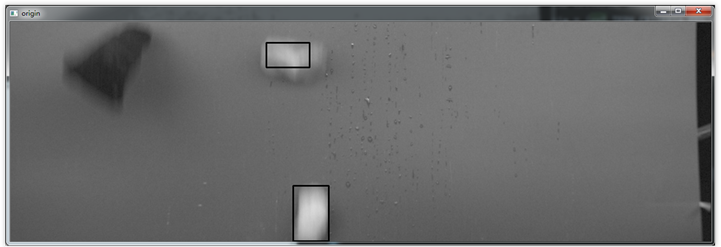Figure 1. Detection of moving ore

4.1. 颜色聚类

4.2. 不同颜色空间颜色聚类分析

4.2.1. RGB和HSI颜色空间的二维颜色分量K-Means聚类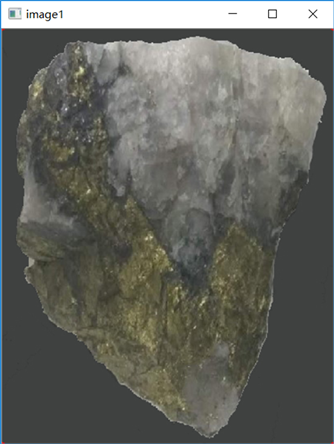Figure 2. Original ore drawing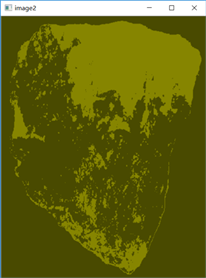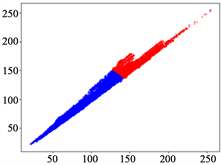(a)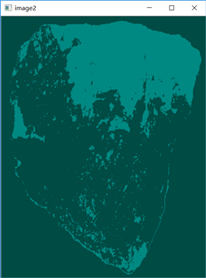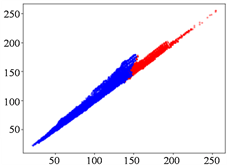(b)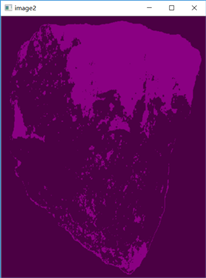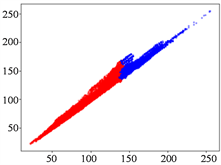(c)

Figure 3. (a) (R,G) Clustering results, (b) (G,B) Clustering results, (c) (R,B) Clustering results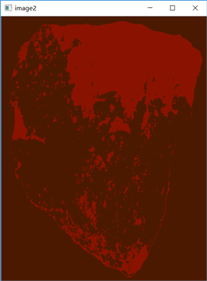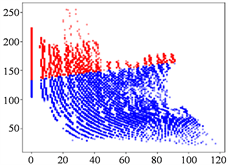(a)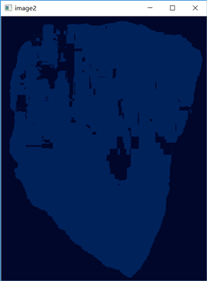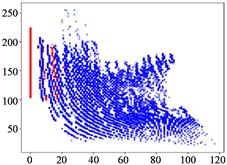(b)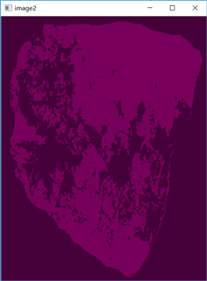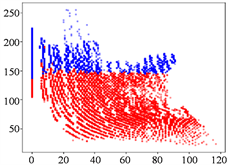(c)

Figure 4. (a) (S,V) Clustering results, (b) (H,S) Clustering results, (c) (H,V) Clustering results

4.2.2. CIELAB颜色空间的二维分量K-Means聚类

5. 算法设计

$G\left(a,b,n\right)=\left\{\begin{array}{l}n,\text{\hspace{0.17em}}\text{\hspace{0.17em}}n\ge {N}_{\mathrm{min}}\\ 0,\text{\hspace{0.17em}}\text{\hspace{0.17em}}\text{ }n<{N}_{\mathrm{min}}\end{array}$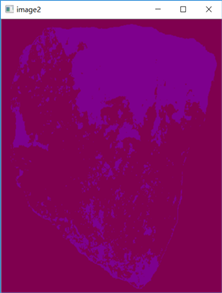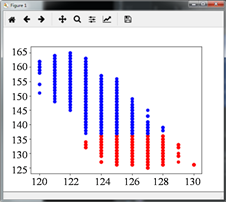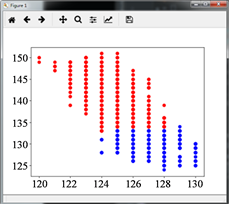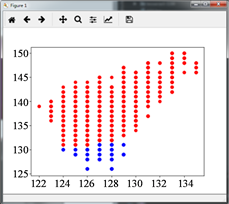Figure 5. (a) (L,a) Clustering results, (b) (L,b) Clustering results, (c) (a,b) Clustering results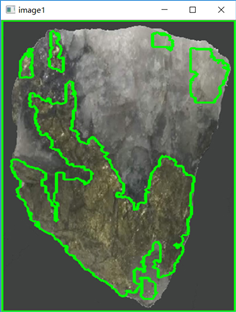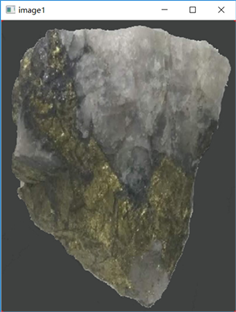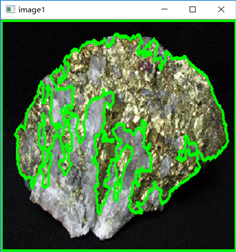Figure 6. Clustering results of different ore images (a, b)

6. 实验结果及分析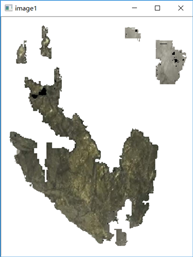Figure 7. The yellow region of ore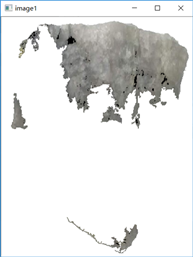Figure 8. Ore white area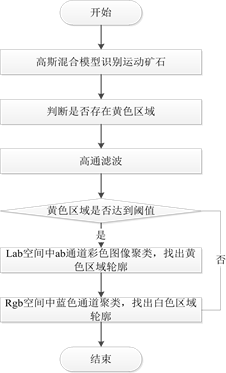Figure 9. Algorithm flow

$\begin{array}{l}检出率=\frac{检测到正确的目标数}{图像中真实目标数}\\ 虚警率=\frac{检测到错误的目标数}{图像中真实目标数}\\ 虚警率=\frac{未检测到的目标数}{图像中真实目标数}\end{array}$

7. 结论

1) 相较与其他视频运动目标检测方法，在钨矿初选过程中混合高斯模型实现多目标运动物体的检测，消耗时间小于其他方法。能够满足工业生产中的实时处理。

2) 在CIELAB颜色空间利用二维颜色分量(a,b)进行矿石图像的K-means颜色聚类分析，使得对于不同照明类型所获得的矿石图像，都可以直接进行处理，所以该算法具有广泛的适用性。

  高玉德. 我国钨矿资源特点及选矿工艺研究进展[J]. 中国钨业, 2016, 31(5): 35-39.  方浩, 艾光华, 刘艳飞. 白钨矿选矿工艺研究现状及发展趋势[J]. 中国钨业, 2016, 31(3): 27-31.  Sun, D.Q., Roth, S. and Black, M.J. (2014) A Quantitative Analysis of Current Practices in Optical Flow Estimation and the Principles behind Them. Interna-tional Journal of Computer Vision, 106, 115-137. https://doi.org/10.1007/s11263-013-0644-x  Rashid, M.E. and Thomas, V. (2016) A Background Foreground Competitive Model for Background Subtraction in Dynamic Background. Procedia Technology, 25, 536-543.  周西汉, 刘勃, 周荷琴. 一种基于对称差分和背景消减的运动检测方法[J]. 计算机仿真, 2005, 22(4): 117-119, 123.  Barnich, O. and Van Droogenbroeck, M. (2011) ViBe: A Universal Background Subtraction Algorithm for Video Sequences. IEEE Transactions on Image Processing, 20, 1709-1724. https://doi.org/10.1109/TIP.2010.2101613  Gao, T., Liu, Z.G., Yue, S.H., et al. (2010) Robust Background Subtraction in Traffic Video Sequence. Journal of Central South University of Technology, 17, 187-195.  Wang, X. and Guo, L. (2012) Highway Traffic Video Detection Method. Journal of Computer Applications, 32, 1585-1588. https://doi.org/10.3724/SP.J.1087.2012.01585  Lezoray, O. and Cardot, H. (2002) Cooperation of Color Pixel Classification Schemes and Color Watershed. IEEE Transactions on Image Processing, 11, 783-789. https://doi.org/10.1109/TIP.2002.800889  Lezoray, O. and Charrier, C. (2009) Color Image Segmentation Using Morphological Lustering and Fusion with Automatic Scale Selection. Pattern Recognition Letters, 30, 397-406. https://doi.org/10.1016/j.patrec.2008.11.005